Balbharti Maharashtra State Board 12th Commerce Book Keeping & Accountancy Solutions Chapter 9 Analysis of Financial Statements Textbook Exercise Questions and Answers.

## Maharashtra State Board 12th Book Keeping & Accountancy Solutions Chapter 9 Analysis of Financial Statements

Objective Questions

A. Select the most appropriate alternative from those given below and rewrite the sentences:

Question 1.
Gross Profit Ratio indicates the relationship of gross profit to the ____________
(a) Net cash
(b) Net sales
(c) Net purchases
(d) Gross sales
(b) Net sales

Question 2.
Current ratio = $$\frac{……………….}{Current liabilities}$$
(a) Quick assets
(b) Quick liabilities
(c) Current assets
(d) None of these
(c) Current assetsQuestion 3.
Liquid assets = ____________
(a) Current assets + Stock
(b) Current assets – Stock
(c) Current assets – Stock + Prepaid Expenses
(d) None of these
(b) Current assets – Stock

Question 4.
Cost of goods sold = ____________
(a) Sales – Gross profit
(b) Sales – Net profit
(c) Sales proceeds
(d) None of these
(a) Sales – Gross profit

Question 5.
Net profit ratio is equal to ____________
(a) Operating ratio
(b) Operating net profit ratio
(c) Gross profit ratio
(d) Current ratio
(a) Operating ratio

Question 6.
The common size statement requires ____________
(a) common base
(b) journal entries
(c) cashflow
(d) current ratio
(a) common base

Question 7.
Bill payable is ____________
(a) long-term loan
(b) current liabilities
(c) liquid assets
(d) net loss
(b) current liabilities

Question 8.
Generally current ratio should be ____________
(a) 2 : 1
(b) 1 : 1
(c) 1 : 2
(d) 3 : 1
(a) 2 : 1Question 9.
From financial statement analysis the creditors are specially interested to know ____________
(a) Liquidity
(b) Profits
(c) Sale
(d) Share capital
(a) Liquidity

B. Give one word/term/phrase for each of the following statements.

Question 1.
The statement showing the profitability of two different periods.
Comparative Income Statement

Question 2.
The ratio measures the relationship between gross profit and net sales.
Gross Profit Ratio

Question 3.
Critical evaluation of financial statement to measure profitability.
Analysis of Financial Statement

Question 4.
A particular mathematical number showing the relationship between two accounting figures.
Ratio

Question 5.
An asset that can be converted into cash immediately.
Liquid AssetQuestion 6.
The ratio measuring the relationship between net profit and ownership capital employed.
ROCE

Question 7.
The statement showing financial position for different periods of the previous year and the current year.
Comparative Balance Sheet

Question 8.
Statement showing changes in cash and cash equivalent during a particular period.
Cash Flow Statement

Question 9.
Activity related to the acquisition of long-term assets and investment.
Financing Activities

Question 10.
The ratio that establishes a relationship between Quick Assets and Current Liabilities.
Liquid Ratio

C. State true or false with reasons.

Question 1.
Financial statements include only the Balance Sheet.
This statement is False.
Financial statements include Balance Sheet and Profit and Loss A/c. This is because financial statements are prepared by business organisations to find out the efficiency, solvency, profitability, growth, strength, and status of the business. For this, they need information from the balance sheet as well as from Profit and Loss A/c.Question 2.
Analysis of financial statements is a tool but not a remedy.
This statement is True.
Based on analysis of the financial statement one can get an idea of the financial strength and weakness of the business. However, based on this one cannot take decisions about the business on various issues. Hence analysis of financial statements is a tool but not a remedy.

Question 3.
Purchase of fixed assets is operating cash flow.
This statement is False.
Purchase of fixed assets is cash flow from investing activities. It is not a day-to-day operations activity like office/selling/distribution finance expenses/activities.

Question 4.
Dividend paid is not a source of funds.
This statement is True.
The dividend is always paid on shares issued by a company as an expense. Shares itself is a source of funds. In payment of dividends, cash goes out from the company. It is an outflow of cash and not a source of funds.

Question 5.
Gross profit depends upon net sales.
This statement is True.
The gross profit ratio discloses the relation between gross profit and total net sales. The gross profit ratio is an income-based ratio, where gross profit is an income. There is a direct relation between net sales and gross profit. Higher the net sales higher the gross profit.Question 6.
Payment of cash against the purchase of stock is the use of funds.
This statement is True.
Cash payment for the purchase of stock is made from cash balance or/and from bank balance which is a part of the business fund. When stock or materials we purchase we use cash for payment.

Question 7.
Ratio Analysis is useful for inter-firm comparison.
This statement is True.
The comparison of the operating performance of a business entity with the other business entities is known as an inter-firm comparison. This ratio analysis assists to know-how and to what extent a business entity is strong or weak as compared to other business entities.

Question 8.
The short-term deposits are considered as cash equivalent.
This statement is True.
The short-term deposits are liquid assets. It means deposits are kept for some period (usually less than one year) and they are kept with an intention to get money quickly as and when required.
They are as good as cash and considered as cash equivalent.

Question 9.
Activity ratios and Turnover ratios are the same.
This statement is True.
Turnover ratio is an efficiency ratio to check how efficiently a company is using different assets to extract earnings from them.
Activity ratios are financial analysis tools used to measure a business’s ability to convert its assets into cash. From both these definitions, we can say that Activity ratios and Turnover ratios are the same.Question 10.
The current ratio measures the liquidity of the business.
This statement is True.
The current ratio shows the relationship between current assets and current liabilities. If the proportion of current assets is higher than current liabilities, the liquidity position of the business entity is considered good. More liquidity means more short-term solvency. From the above, it is proved that the current ratio measures the liquidity of the business.

Question 11.
Ratio analysis measures profitability efficiency and financial soundness of the business.
This statement is True.
With the help of profitability ratios (Gross profit, Net profit, and Operating profit) one can get the idea of profitability efficiency of the firm, and with the help of liquidity ratios (Current ratio and liquid ratio) one can get the idea of solvency or financial soundness of the business.

Question 12.
Usually, the current ratio should be 3 : 1.
This statement is False.
Usually, the current ratio should be 2 : 1. It means current assets are double of current liabilities. It shows the short-term solvency of business enterprises.

D. Answer in one sentence only.

Question 1.
Mention two objectives of the comparative statement.
Objectives of comparative statements are:

• Compare financial data at two points of time and
• Helps in deriving the meaning and conclusions regarding the changes in financial positions and operating results.

Question 2.
State three examples of cash inflows.
Examples of cash inflows are:

• Sale of asset/investmentQuestion 3.
State three examples of cash-out flows.
Examples of cash outflows are:

• Interest paid
• Loss on sale of an asset
• Dividend paid
• Repayment of short-term borrowings.

Question 4.
Give the formula of Gross Profit Ratio.
Gross profit ratio = $$\frac{\text { Gross profit }}{\text { Net sales }} \times 100$$
Where Gross profit = Net sales – Cost of goods sold.
Cost of goods sold = Opening stock + Purchase – Purchase return + Direct expense – Closing stock
Net sales = Sales – Sales return.

Question 5.
Give the formula of Gross profit.
Gross profit = Net sales – Cost of goods sold.
Cost of goods sold = Opening stock + Purchase – Purchase return + Direct expense – Closing stock
Net sales = Sales – Sales return.

Question 6.
Give any three examples of current assets.
Cash or cash equivalent short-term lending and advances, expenses paid in advance, taxes paid in advance, etc. are examples of current assets.

Question 7.
Give the formula of the current ratio.
Current ratio = $$\frac{\text { Current assets }}{\text { Current liabilities }}$$

Question 8.
Give the formula of quick assets.
Quick assets = Current assets – (Stock + Prepaid expense)Question 9.
State the formula of Cost of goods sold.
Cost of goods sold = Opening stock + Purchase – Purchase return + Direct expense – Closing stock

Question 10.
State the formula of Average stock.
Average stock = $$\frac{\text { Opentng stock of goods }+\text { Closing stock of goods }}{2}$$

Practical Problems

Question 1.
From the Balance Sheet of Amar Traders as of 31st March 2018 and 31st March 2019 prepare a Comparative Balance Sheet.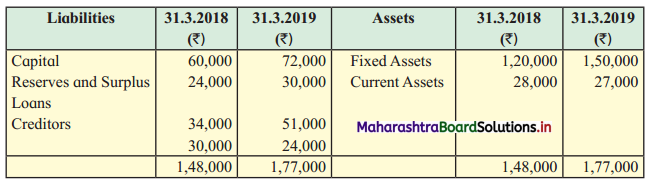Solution:
Comparative Balance Sheet of Amar Traders as of 31st March 2018 and 31st March 2019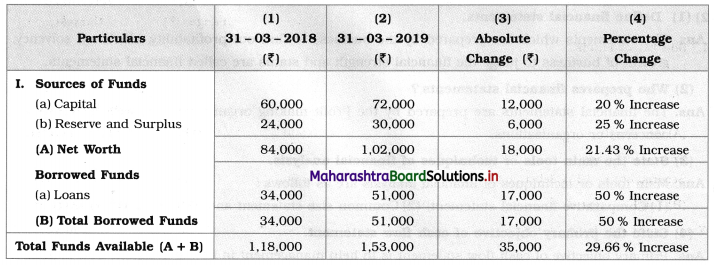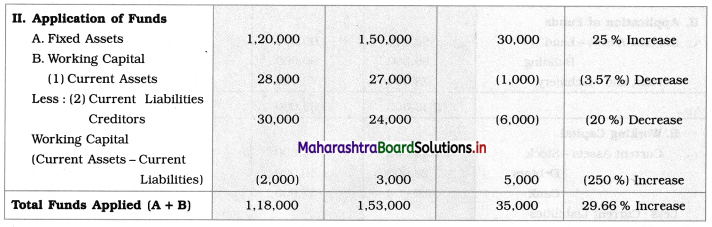Percentage change = $$\frac{\text { Amount of absolute change }}{\text { Amount of previous year }} \times 100$$

Question 2.
From the Balance Sheet of Alpha Limited prepare a Comparative Balance Sheet as of 31st March 2018 and 31st March 2019:
Balance Sheet as of 31st March 2018 and 31st March 2019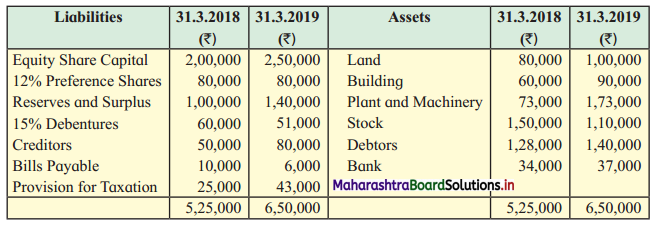Solution:
Comparative Balance Sheet of Alpha Limited as of 31st March 2018 and 31st March 2019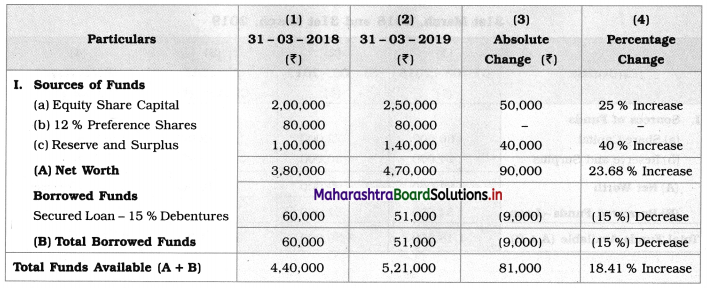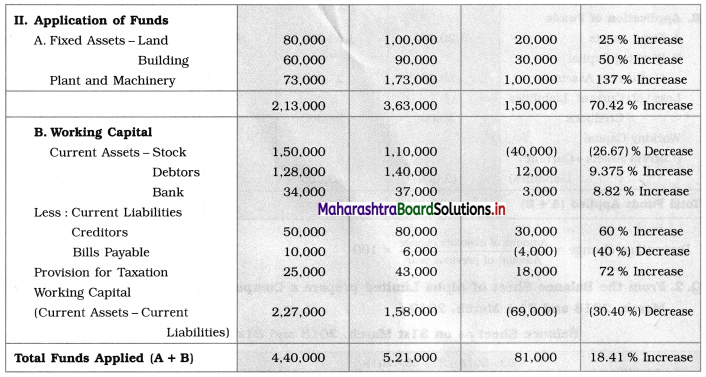Question 3.
Prepare Comparative Balance Sheet for the year ended 31-3-18 and 31-3-19. Assets & Liabilities as follows: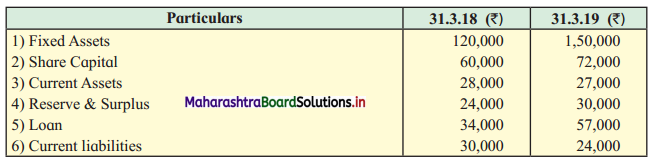Solution:
Comparative Balance Sheet as of 31st March 2018 and 31st March 2019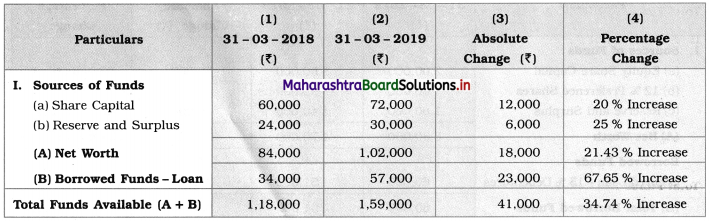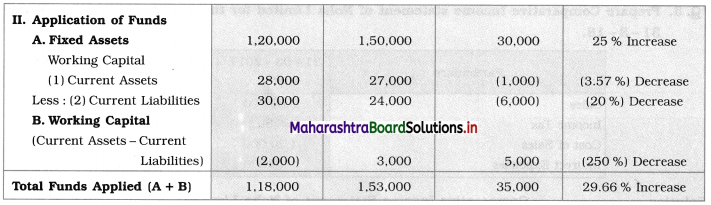Question 4.
Prepare Comparative Balance Sheet for the year ended 31-3-17 and 31-3-18.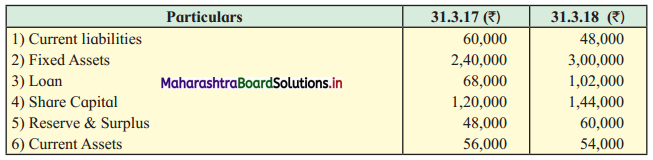Solution:
Comparative Balance Sheet as of 31st March 2017 and 31st March 2018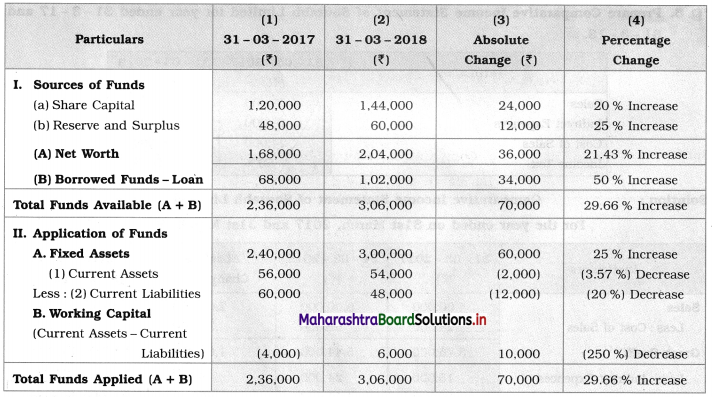Question 5.
Prepare Comparative Income statement of Noha Limited for the year ended 31-3-17 and 31-3-18.Solution:
Comparative Income Statement of Noha Limited
For the year ended 31st March 2017 and 31st March 2018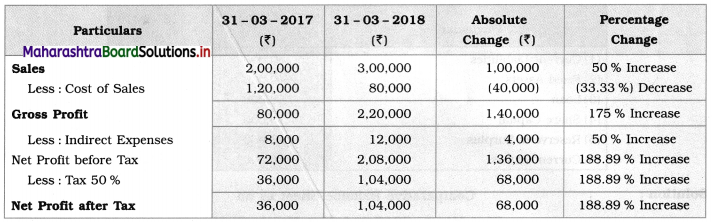Question 6.
Prepare Comparative Income Statement of Sourabh Limited for years ended 31-3-17 and 31-3-18.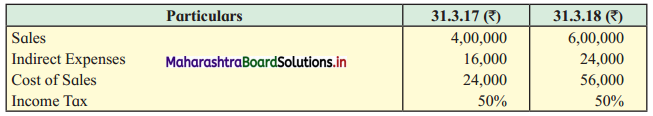Solution:
Comparative Income Statement of Sourabh Limited
For the year ended on 31st March 2017 and 31st March 2018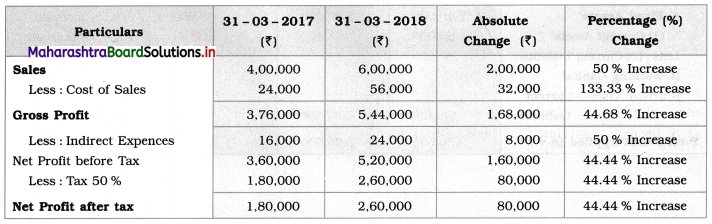Question 7.
Following is the Balance Sheet of Sakshi Traders for the year ended 31-3-17 and 31-3-18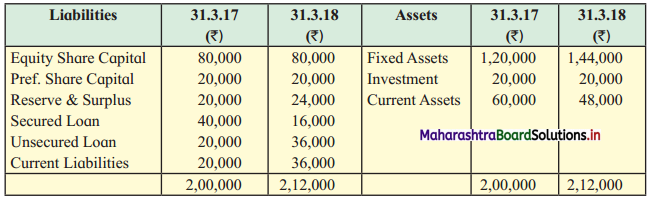Prepare Common Size Balance Sheet for the years 31-03-17 and 31-03-18.
Solution:
Common Size Statement of Balance Sheet of Sakshi Traders as of 31st March 2017 and 31st March 2018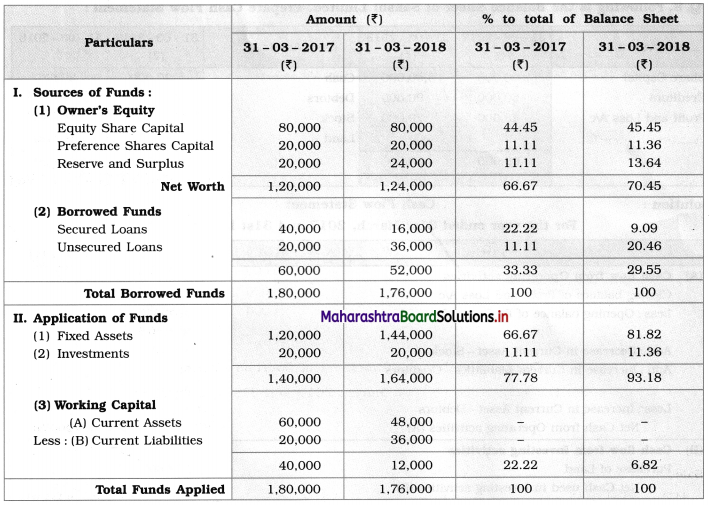Note: Taking Total borrowed funds and Tota Funds applied as a base (100), Calculation is done.

Question 8.
Prepare Common Size Income Statement for the year ended 31-3-17 and 31-3-18.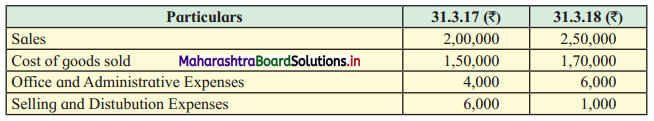Solution:
Common Size Statement for the year ended on 31st March 2017 and 31st March 2018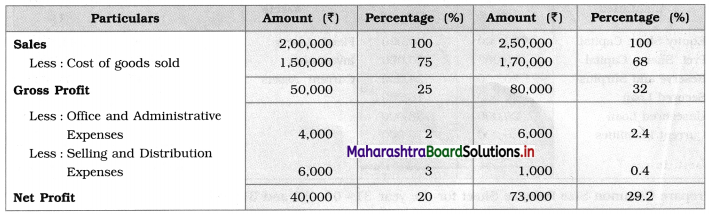Note: Taking the amount of sales as base (100) other percentage figures are calculated.

Question 9.
Following is the Balance Sheet of Sakshi Limited. Prepare Cash Flow Statement: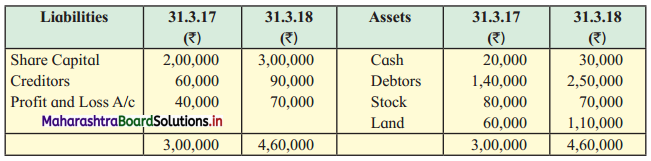Solution:
Cash Flow Statement
For the year ended 31st March 2017 and 31st March 2018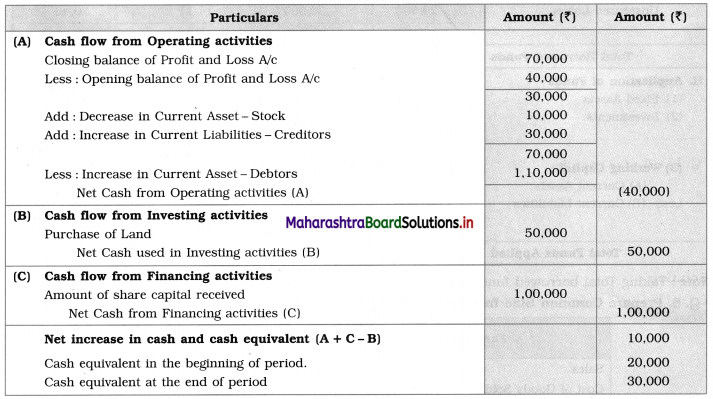Question 10.
From the following Balance Sheet of Konal Traders prepare a Cash Flow Statement.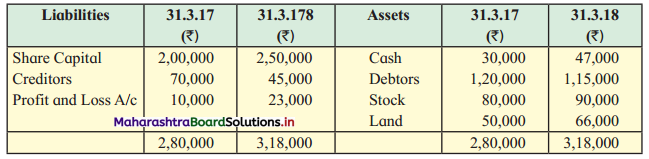Solution:
Cash Flow Statement
For the year ended on 31st March 2017 and 31st March 2018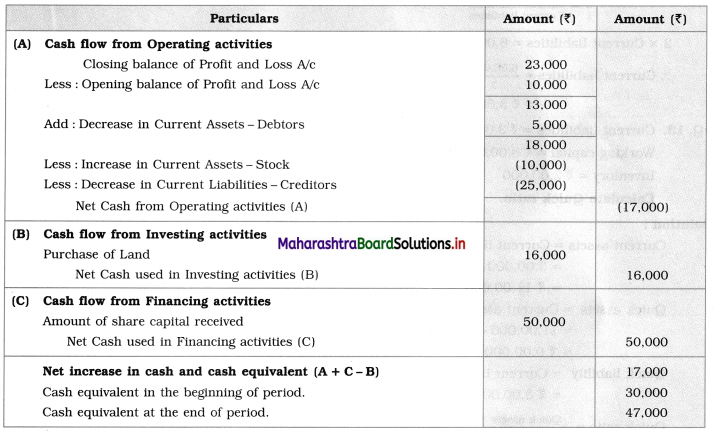Question 11.
A company had following Current Assets and Current Liabilities:
Debtors = ₹ 1,20,000
Creditors = ₹ 60,000
Bills Payable = ₹ 40,000
Stock = ₹ 60,000
Loose Tools = ₹ 20,000
Bank Overdraft = ₹ 20,000
Calculate Current ratio.
Solution:
1. Current Assets = Debtors + Stock + Loose Tools
= 1,20,000 + 60,000 + 20,000
= ₹ 2,00,000

2. Current liabilities = Creditors + Bills payable + Bank overdraft
= 60,000 + 40,000 + 20,000
= ₹ 1,20,000

3. Current ratio = $$\frac{\text { Current assets }}{\text { Current liabilities }}$$
= $$\frac{2,00,000}{1,20,000}$$
= $$\frac{5}{3}$$
= 5 : 3

Question 12.
Current assets of company ₹ 6,00,000 and its Current ratio is 2 : 1. Find Current liabilities.
Solution:
Current ratio = $$\frac{\text { Current assets }}{\text { Current liabilities }}$$
$$\frac{2}{1}=\frac{6,00,000}{\text { Current liabilities }}$$
2 × Current liabilities = 6,00,000 × 1
Current liabilities = $$\frac{6,00,000}{2}$$ = ₹ 3,00,000Question 13.
Current liabilities = ₹ 3,00,000
Working capital = ₹ 8,00,000
Inventory = ₹ 2,00,000
Calculate Quick ratio.
Solution:
Current assets = Current liabilities + Working capital
= 3,00,000 + 8,00,000
= ₹ 11,00,000
Quick assets = Current assets – Inventory
= 11,00,000 – 2,00,000
= ₹ 9,00,000
Quick liability = Current liabilities – Bank O/D = ₹ 3,00,000
Quick ratio = $$\frac{\text { Quick assets }}{\text { Quick liabilities }}$$
= $$\frac{9,00,000}{3,00,000}$$
= $$\frac{3}{1}$$
= 3 : 1

Question 14.
Calculate Gross Profit ratio
Sales = ₹ 2,70,000
Net purchases = ₹ 1,50,000
Sales Ratio = ₹ 20,000
Closing Stock = ₹ 25,000
Operating Stock = ₹ 45,000
Solution:
Net sales = Sales – Sales return
= 2,70,000 – 20,000
= ₹ 2,50,000
Cost of goods sold = Opening stock + Net purchase – Closing stock
= 45,000 + 1,50,000 – 25,000
= ₹ 1,70,000
Gross profit = Net sales – Cost of goods sold
= 2,50,000 – 1,70,000
= ₹ 80,000
Gross Profit ratio = $$\frac{\text { Gross profit }}{\text { Net sales }} \times 100$$
= $$\frac{80,000}{2,50,000} \times 100$$
Gross profit ratio = 32%

Question 15.
Calculate Net Profit ratio from the following:
Sales = ₹ 3,80,000
Cost of goods sold = ₹ 2,60,000
Indirect expense = ₹ 60,000
Solution:
Sales = ₹ 3,80,000
Less: Cost of goods sold = ₹ 2,60,000
Gross profit = ₹ 1,20,000
Less: Indirect expense = ₹ 60,000
Net profit = ₹ 60,000
Net profit ratio = $$\frac{\text { Net profit }}{\text { Sales }} \times 100$$
= $$\frac{60,000}{3,80,000} \times 100$$
= 15.79%Question 16.
Calculate Operating ratio:
Cost of goods sold = ₹ 3,50,000
Operating expense = ₹ 30,000
Sales = ₹ 5,00,000
Sales return = ₹ 30,000
Solution:
Net sales = Sales – Sales return
= 5,00,000 – 30,000
= ₹ 4,70,000
Operating ratio = $$\frac{\text { Cost of goods sold }+\text { Operating expense }}{\text { Net sales }} \times 100$$
= $$\frac{3,50,000+30,000}{4,70,000} \times 100$$
= $$\frac{3,80,000}{4,70,000} \times 100$$
= 80.85%

Question 17.
Calculate Current ratio.
1. Current assets = ₹ 3,00,000
2. Current liabilities = ₹ 1,00,000
Solution:
Current ratio = $$\frac{\text { Current assets }}{\text { Current liabilities }}$$
= $$\frac{3,00,000}{1,00,000}$$
= $$\frac{3}{1}$$
= 3 : 1